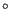#### catnodes.f

In the smoothing procedure the position of a node i is replaced by a weighted average of the position of its neighbors. In principal, all nodes belonging to the ball of node i (the ball of a node is by definition the set of element to which this node belongs ) are neighbors. However, for some nodes this set is further restricted. In general, a node j belonging to the ball of node i is a neighbor of node i if:

• node i is internal OR
• node is is external, node j is external and no sharp edges are attached to node i OR
• node i is external, node j is external and the edge connecting both is sharp.

Thus, the neighbors of an external node must also be external, else the weighted average “pulls” the node into the interior of the body, which is not beneficial. For the storage of the neighbors fields iponn(*) and inn(2,*) are used. The neighbors of node i are calculated in catnodes.f (“catalogue nodes”) and are stored in inn(1,iponn(i),), inn(1,inn(2,iponn(i)), inn(1,inn(2,inn(2,iponn(i))), ... until inn(2,inn(2,.....))))=0. In this context also field idimsh(*) is used: idimsh(i) is the number of sharp edges connected to node i. Sharp edges are by definition external edges in the unrefined mesh the normals on the adjacent faces of which make an angle exceeding 0.0464(determined in checksharp.f).